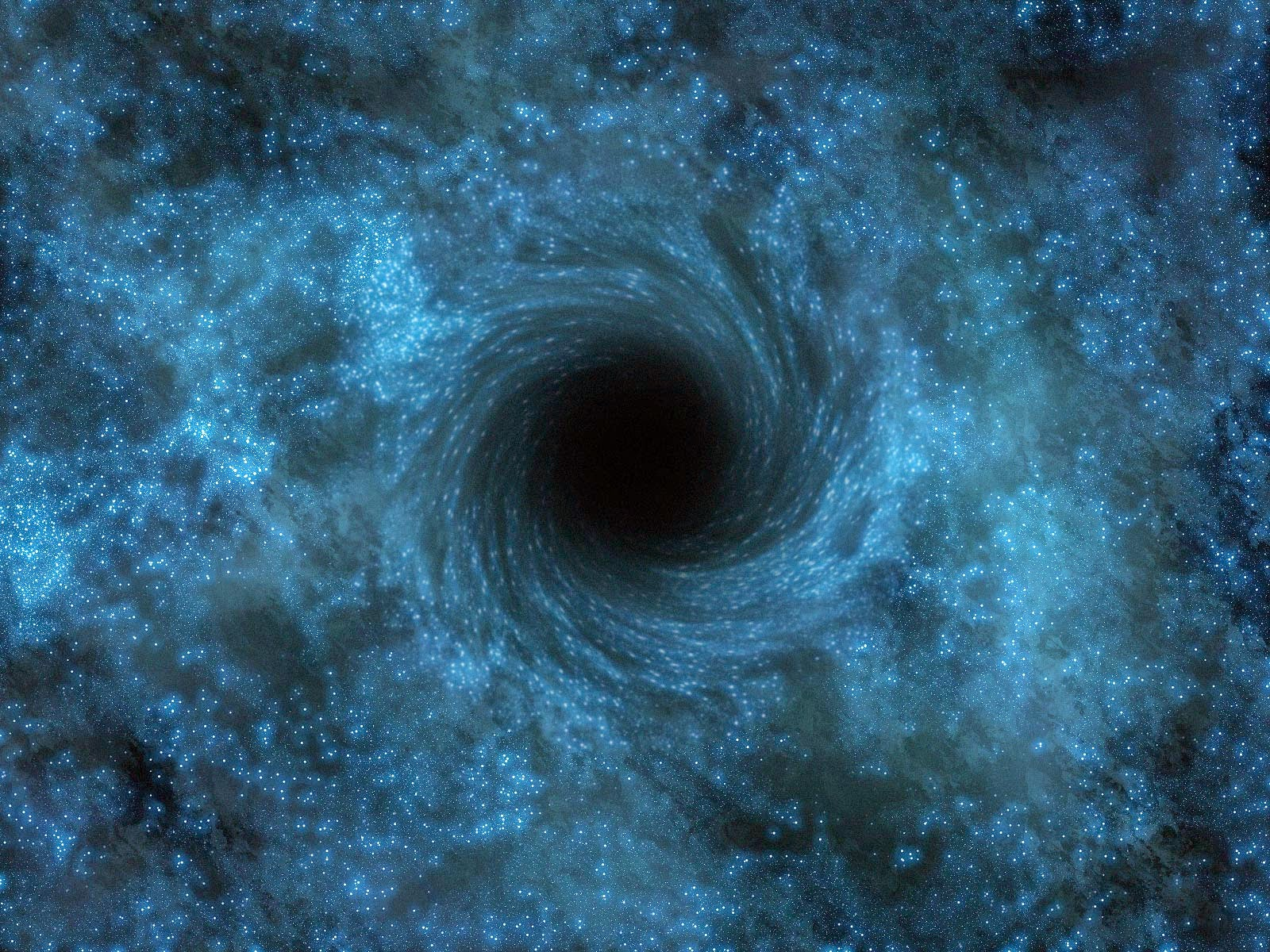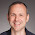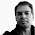## Saturday, May 10, 2014

### The science of citations. (Also: black holes, again).

If the title of this blog post made you click on it, I owe you an apology. All others, that is, those who got here via perfectly legitimate reasons, such as checking this blog with bated breath every morning--only to be disappointed yet again ... bear with me.

Here I'll tell you why I'm writing about the same idea (concerning, what else, the physics of black holes) for the fourth time.

You see, an eminent scientist (whose name I'm sure I forgot) once told me that if you want to get a new idea noticed among the gatekeepers of that particular field, you have to publish it five times. The same idea, he said, over and over.

I'm sure I listened when he said that, but I'm equally sure that, at the time (youthful as I was), I did not understand. Why would the idea, if it was new (and let's assume for the sake of the present argument) worthy of the attention and study of everyone working in the field, not take hold immediately?

Today I'm much wiser, because now I can understand this advice as being a perfectly commonplace application of the theory of fixation of beneficial alleles in Darwinian evolution.

Stop shaking your head already. I know you came here to get the latest on the physics of black holes. But before I get to that (and I will), this little detour on how science is made--via the mathematics of population genetics no less--should be worth your time.

In population genetics (the theory that deals with how populations and genes evolve), a mutation (that is, a genetic change) is said to have "gone to fixation" (or "fixated") if everybody in the population carries this mutation. This implies that all those that ever did NOT carry that mutation are extinct at the time of fixation. From the point of view of scientific innovation, fixation is the ultimate triumph of an idea: if you have achieved it, then nobody who ever did NOT believe you is dead as a doornail.

Fixation means you win. What do you have to do to achieve that?

Of course, in reality such "fixation of ideas" is never fully achieved, as in our world there will always be people who doubt that the Earth is round, or that the Earth revolves around the Sun, or that humans are complicit in the Earth's climate change. I will glibly ignore these elements. (As I'm sure you do too). Progress in science is hard enough even in the absence of such irrationality.

When a new idea is born, it is a fragile little thing. It germinates in the mind of its progenitor first tentatively, then more strongly. But even though it may one day be fully formed and forcefully expelled by its creative parent into the world of ideas, the nascent idea faces formidable challenges. It might be better than all other ideas in hindsight. But today is not hindsight: here, now, at the time of its birth, this idea is just an idea among many, and it can be snuffed out at a moment's notice.

The mathematics of population genetics teaches us that much: to calculate the probability of fixation of a mutation, you start by calculating its probability to go extinct. Let's think of a new idea as the mutation of an old idea.

What does it mean for an idea to go extinct? If we stick to ideas published in the standard technical literature, it means for that idea (neatly written into a publication) to never be cited because citations are, in a very real way, the offspring your idea germinates. You remember Mendel, the German-speaking Silesian monk who discerned the mathematical laws of inheritance at a time when Darwin was still entirely in the dark about how inheritance works?Gregor Mendel (1822-1844) Source: Wikimedia
(Incidentally, my mother was born not far from Mendel's birthplace.)

Well, Mendel's idea almost got extinct. He published his work about the inheritance of traits in 1866, in the journal Verhandlungen des naturforschenden Vereins Brünn. Very likely, this is the most famous paper to ever appear in this journal, and to boot it carried the unfortunate title "Versuche über Pflanzenhybriden" (Experiments on Plant Hybridization). Choosing a bad title can really doom the future chances of your intellectual offspring (I know, I chose a number of such unfortunate titles), and so it was for Mendel. His paper was classified under "hybridization" (rather than "inheritance"), and lay dormant for 35 years. Imagine your work laying dormant for 35 years. Now stop.

The idea of Mendelian genetics was, for all purposes, extinct. But unlike life forms, extinct ideas can be resurrected. As luck would have it, a Dutch botanist by the name of de Vries conducted a series of experiments hybridizing plant species in the 1890s, and came across Mendel's paper. While clearly influenced by that paper (because de Vries changed his nomenclature to match Mendel's), he did not cite him in his 1900 paper in the French journal Comptes Rendus de l'Académie des Sciences. And just like that, Mendel persisted in the state of extinction once more.

Except that this time, the German botanist and geneticist Carl Correns came to Mendel's rescue. Performing experiments on the hawkweed plant, he rediscovered Mendel's laws, and because he knew a botanist that had corresponded with Mendel, he was familiar with that work. Correns went on to chastize de Vries for not acknowledging Mendel, and after de Vries starting citing him, the lineage of Mendel's idea was established forever.

You may think that this is an extraordinary sequence of events but I can assure you, this is by no means an exception. Science does not progress in a linear predictable fashion, mostly because the agents involved (namely scientists) are not exactly predictable machines. They are people. Even in science, the fickle fortunes of chance reign supremely.

But chance can be described mathematically. Can we calculate the chance that a paper will become immortal?

Let us try to estimate the likelihood that a really cool paper (that advances science by a great deal) becomes so well-known that everybody in the field knows it (and therefore cites it, when appropriate). Let's assume that said paper is so good that it is better than other papers by a factor $1+s$, where $s$ is a positive fraction ($s=0.1$ if the paper is 10% better than the state of the art, for example).  Even though this paper is "fitter"--so to speak--then all the other papers it competes with for attention, it is not obvious that it will become known instantly, because whether someone picks up the paper, reads it, understands it, and then writes a new paper citing it, is a stochastic (that is, probabilistic) process. So many things have to go right.

We can model these vagaries by using what is known as a Poisson process, and calculate the probability $p(k)$ that a paper gets $k$ citations
$$p(k)=\frac{e^{-(1+s)}(1+s)^k}{k!}. (1)$$.
Poisson processes are very common in nature: any time you have rare events, the cumulative distribution (of how many of these rare events ended up occurring) is distributed like (1).

Note that because the mean number of citations of the paper (the average of the distribution), is $1+s$ and thus larger than one, and if we moreover assume that the papers that cite your masterpiece "inherit" the same advantage (because they also use that cool new idea) then in principle the number of citations of this paper should explode exponentially with time. But that is not a guarantee.

Let's calculate the probability $P$ that the paper actually "takes off". In fact, it is easier to calculate the probability that it does NOT take off: that it languishes in obscurity forever. This is $1-P$. (Being summarily ignored is the fate of several of my papers, and indeed almost all papers everywhere).

This (eternal obscurity) can happen if the paper never gets cited in the first place (given by $p(0)$ in Eq. (1) above), plus the probability that it gets cited once (but the citing paper itself never gets cited: $p(1)(1-P)$) plus the probability that it gets cited twice, but BOTH citing papers don't make it:  $p(2)(1-P)^2$, and so on.

Of course, the terms with higher $k$ become more and more unlikely (because when you get 10 citations, say, it is quite unlikely that all ten of them will never be cited). We can calculate the total "extinction probability" as an infinite sum:
$$1-P=\sum_{k=0}^{\infty} p(k)(1-P)^k, (2)$$
but keep in mind that the sum is really dominated by its first few terms. Now we can plug formula (1) into (2), do a little dance, and you get the simple looking formula
$$1-P=e^{-(1+s)P} . (3)$$
That looks great, because you might think that we can now easily calculate $P$ from $s$. But not so fast.

You see, that Eq. (3) is a recursive formula. It calculates $P$ in terms of $P$. You are of course not surprised by that, because we explicitly assumed that the likelihood that the paper never becomes well known depends on the probability that the few papers that cite it are ignored too. Your idea becomes extinct if all those you ever influenced are reduced to silence, too.

While the formula cannot be solved analytically, you can calculate an approximate form by assuming that the paper is great but not world shaking. Then, the probability $P$ that it will become well-known is small enough that we can approximate the exponential function in (3) as
$$e^{-(1+s)P}\approx 1-(1+s)P+\frac12(1+s)^2P^2,$$
that is, we keep terms up to quadratic in $P$. And the reason why $P$ is small is because the advantage $s$ isn't fantastically large either. So we'll ignore everything that has powers of $s^2$ or higher in $s$.

Then Eq. (3) becomes
$$1-P\approx 1-P-sP+\frac12 P^2 +sP^2,$$ or
$$P=\frac{2s}{1+2s}. (4)$$
What we derived here is actually a celebrated result from population genetics, due to the mathematical biologist J. B. S. Haldane.J. B. S. Haldane (1892-1964)
If you're not already familiar with this chap, spend a little time with him. (Like, reading about him on Wikipedia). He would have had a lot to complain about in this century, I'm thinking.

So what does this result teach us? If you take equation (4) and expand it to lowest order in $s$ (this means, you neglect all terms of order $s^2$), you get the result that is usually associated with Haldane, namely that the probability of fixation of an allele with fitness advantage $s$ is
$$P(s)=2s. (5)$$.
Here's what this means for us: if an idea is ten percent better than any other idea in the field, there is only a 20% chance that it will get accepted (via the chancy process of citation).

These are pretty poor odds for an obviously superior idea! What to make of that?

The solution to this conundrum is precisely the advice that the senior scientist imparted on me!

Try, try, again.

I will leave it to you, dear reader, to calculate the odds of achieving "fixation" if you start the process five independent times. (Bonus points for a formula that calculates the probability of fixation for $n$ independent attempts at fixation).

So now we return to the first question. Why am I writing a fourth paper on the communication capacity of black holes (with a fifth firmly in preparation, I might mention)?

Because the odds that a single paper will spread a good idea are simply too small. Three is good. Four is better. Five is much better.

And in case you are trying to keep track, here is the litany of tries, with links when appropriate:

(1) C. Adami and G. Ver Steeg. Class. Quantum Gravity 31 (2014) 075015  blogpost
(2) C. Adami and G. Ver Steeg. J. Phys. A 48 (2015) 23FT01 blogpost
(3) K. Brádler and C. Adami, JHEP 1405 (2014) 095 (also preprint arXiv:1310.7914) blogpost
(4) K. Brádler and C. Adami, arXiv preprint arXiv:1405.1097. Read below.

The last paper, entitled "Black holes as bosonic Gaussian channels" was written with my colleague Kamil Bradler (just as the paper that was the object of my most watched blog post to-date). In there we show--again--what happens to quantum information interacting with a black hole, only that in paper (3) we could make definitive statements only about two very extreme black hole channels: those that perfectly reflect information, and those that perfectly absorb. While we cannot calculate all capacities in the latest paper (4), we can do this now as accurately as current theory allows, using the physics of "Gaussian states", rather than the "qubits" that we used previously. Gaussian states are quantum superpositions of particles that are such that if you would measure them, you get a distribution (of the number of measured particles) that is Gaussian. Think of them as wavepackets, if you wish. They are very convenient in quantum optics, for example. And they turn out to be very convenient to describe black hole channels too.

So we are getting the message out  one more time. And the message is loud and clear: Black holes don't mess with information. The Universe is fine.Nothing to see here. Move along.

#### 2 comments:

1.I think you meant "earth revolves around the sun."

2.Fixed. I always mix those up :-)